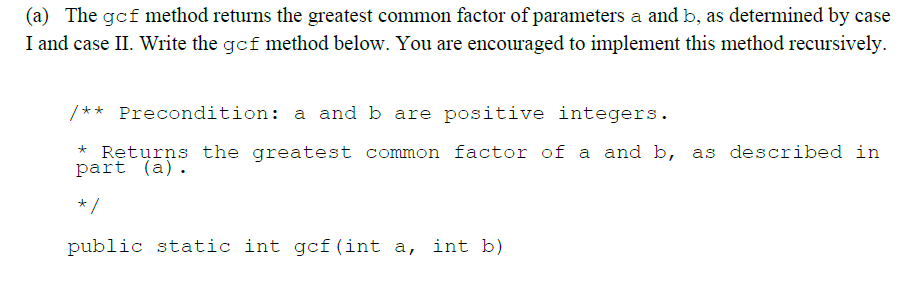# Divisibility and Greatest Common Divisors DRAFT

## Introduction

Yesterday morning, our AP CSA teacher, the good old Bharati, assigned us an College Board's official free response question. When we were asked to recursively find the greatest common divisor of two integers, I was sated, in that in such a class for dummies students finally have chance to learn about one of the few classic and ingenious algorithms that evolved before the Common Era (AD).College Board's FRQ Progress Check 10

This excitement, however, was washed away this morning when we received the grading guideline. In it, College Board did not provide a proof of correctness of its solution that uses Euclidean algorithm, but merely threw readers a block of code, as if saying, "Here you go, problem solved".

## Divisibility Relation

Before getting started, we shall review divisibility among integers mostly to set some notations and to indicate its properties

Definition 2.1. When $a$ and $b$ are integers, we say $a$ divides $b$ if $b=ak$ for some $k \in \Z$. We then write $a \mid b$ (read as "$a$ divides $b$").

The set of integers is denoted $\Z$, from the German word Zahl = number.

Example 2.2. We can have $2 \mid 6$ (because $6 = 2 \cdot 3$), $4 \mid (-12)$, and $5\mid 0$. We also have $\pm 1 \mid a$ for every $a \in \Z$. However, $6$ does not divides $3$ and 0 certainly does not divides $5$.

Divisibility is a relation, much like inequalities, instead of an operator. In particular, the relation $2 \mid 6$ does not spill out the number $3$; equivalently, $2 < 6$ should not be confused with $6-2$.

Also note that divisibility is not symmetric: if $a\mid b$, it is usually not true that $b \mid a$, so you should never confuse the roles of $a$ and $b$ in the relation: $2 \mid 6$ but $6 \nmid 2$.

Remark 2.3. One should strictly follow the definition in Definition 2.1, rather than following the intuition "$\frac a b$ is an integer". Although they essentially mount the same (exception: $0 \mid 0$ but $\frac 0 0$ is undefined), thinking about divisibility in terms of ratios can easily screw up your understanding of divisibility of in other scenarios in algebra. Nonetheless, it is best to regard Definition 2.1.

Next I shall throw a couple of theorems about divisibility that are no more than simple applications of the difinition. They all make intuitive sense.

Theorem 2.4. Let $a,b \in \Z$ with $a \mid b$, then $a\mid bc$ for any $c \in \Z$.

Proof. By definition, we have $b = ak$ for some $k \in \Z$. Thus $bc = (ak)c = a(kc)$ and $kc \in \Z$, which gives the claim. $\square$

Theorem 2.5. If $a \mid b$ and $b \mid c$ then $a \mid c$.

Proof. Now we have $b=ak$ and $c=bl$ for some $k,l \in \Z$. Then $c = bl = (ak)l =a(kl)$ and $kl \in \Z$, so $a\mid c$. $\square$

Theorem 2.6. If $a\mid b$ and $a\mid c$ then $a\mid (br+cs)$ for every $r,s \in \Z$.

Proof. We have $b = ak$ and $c = al$ for some $k,l \in \Z$. Then

$br + cs = (ak)r + (al)s = a(kr+ls)$

and $kr + ls \in \Z$, so $a\mid (br+cs)$. $\square$

In the language of linear algera, Theorem 2.6 says any factor of two integers is also a factor of any $\Z$-linear combination of two integers. To avoid silly errors, keep in mind the following false implications: generally

$a \mid bc \not\Rightarrow a \mid b\ \textrm{or}\ a\mid c$

(for instance $6 | (4 \cdot 9)$ but 6 divides neither 4 or 9) and

$a \mid b^n \not\Rightarrow a \mid b$

(for instance $12 \mid 6^6$ but $12$ does not divide $6$).

## Greatest Common Divisors

For two nonzero integers $a$ and $b$, their greatest common divisor is the largest integer which is a factor of both of them. It is denoted $(a,b)$. For instance, $(12, 18) = 6$ and $(-9,15)=3$. Do not confuse our usage of parentheses in $(a,b)$ with the notation for open intervals in calculus. The number $1$ is always a common divisor, and it is the greatest common divisor exactly when $a$ and $b$ are relatively prime.

The naive approach of finding the greatest common divisor of two integers in to factor each into primes and extract the greatest common divisor from the prime power factors that appear.

Example 3.1. Consider $a = 19088597$ and $b = 39083$. Since

$19088597 = 11^2 \cdot 19^3 \cdot 23,\ 39083 = 11^2 \cdot 17 \cdot 19,$

we have $(19088597, 39083) = 11^2 \cdot 19 = 2299$.